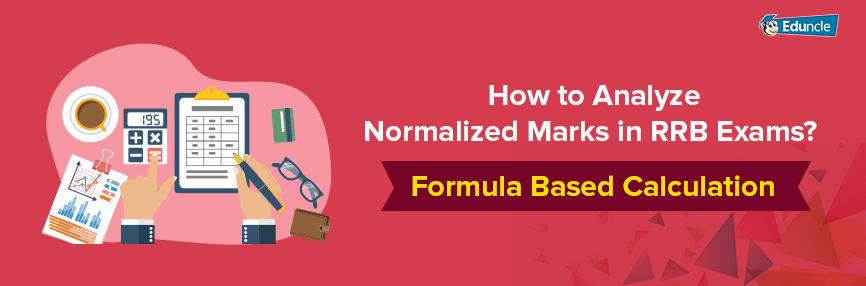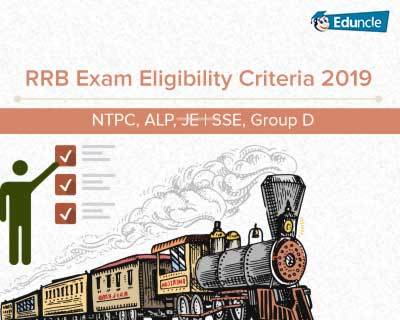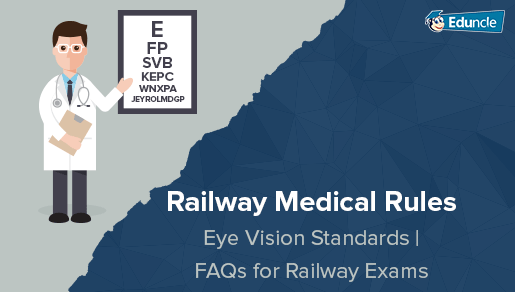RRB FollowRavi @Eduncle posted an Article
August 28, 2019 • 12:36 pm

# How to Analyze Normalized Marks in RRB Exam? Formula Based CalculationWith over 80 lakh students appearing in Railway Recruitment Exams every year, the RRB has become the most sought-after destination for job seekers.

The students can appear in RRB Exams right from their school days. The exams like – RRB ALP, RRB JE|SSE, RRC Group D & NTPC give students an opportunity to make a successful career in the Government Sector.

When such vast number of candidates are taking part in RRB Exams, the number of vacant seats is decreasing day by day. In results, the competition is getting stiffer and qualifying these exams is becoming very challenging.

To identify the best talent through effective evaluation, the exam convener authorities use a formula called as ‘Marks Normalization’. This formula helps examiners to do fair marking based on the paper’s difficulty level.

If you’re getting confused about the term ‘Normalization’, you can read the entire blog and find out how authorities calculate the Railway Exams Marks.

## What is Normalization Marks Procedure in RRB Exams? Get the Complete Knowledge from Here!

In RRB ALP/NTPC/JE/Group D,  Normalization Marks Scheme is used for ensuring that aspirants neither benefited nor deprived by the difficulty level of examination.

As we know that, RRB conducts examinations on multiple dates and shifts, it is impossible to have two questions sets of the same difficulty level in a single shift. So, the authorities conduct the exams on different dates and shifts with a different RRB Question Paper.

Due to this, some of the candidates end up attempting relatively tougher set to other sets.

The aspirants who sit in the comparatively tough examination gets lower marks as compared to those who attempt the easier one.

You might be thinking that despite such complexity, how RRB calculates the marks announce the results!

Let's explore the whole cycle to calculate the marks. Scroll down the blog and get the relevant knowledge for the same.

## How to Calculate Normalized Marks in RRB ALP/NTPC/JE/Group D – Get the Complete Procedure from Here!

We hope that the above-mentioned information is enough to understand the meaning of normalized marks. Now you should also know how these marks are being made uninformed.

Below, we have described the complete procedure to calculate the normalized marks. Have a look!

### Terms to be Considered to Proceeding Further:

There are some basic terms which should be known by you to calculate the normalized marks in RRB Exam which are discussed below

Normalization procedure is a Scientific and Statistical Process. The process is not for awarding the grace marks.

The process is completely based on the statistical parameters calculated on the basis of performance of the candidate in the RRB Exam in all sessions.

The normalization procedure will be totally based on the raw score of candidates. Raw Score is known as the initial stage of the calculation of marks. These are the scores which are obtained by the aspirants by answering correctly in each section of the Exam. These are the scores calculated after applying the penalty for wrong answers.

Example – If the negative marking is 1/3 and candidate answered 70 questions, out of which 12 were wrong, then the Raw score will be 70-12-12*(1/3) = 54, Here 54 are Raw Marks.

The statistical parameters of a session e.g. mean marks and standard deviation of marks scored by the aspirants in the session of RRB Exam are analysed and totally based on the calculated values of all these parameters a session is taken as the base session.

The base session is taken to equalize the level of difficulty of each session.

Among the sessions of RRB Exams that having 70% or above the average attendance of all the session, the mean of the raw score with the maximum value will be taken as the base session.

Here you can also Download the RRB Previous Year Papers to get the excellence!

## RRB Normalization Process – Check Out the Formula

Railway has adopted a prefixed formula to calculate the normalized marks. The Raw Marks & Average Marks play the major role in this formula.

This formula is also known as Normalized Marks Calculator.

Xn= (S2/S1) (X-Xav) + Yav

Xn = Normalised Score of a Candidate

S2 = Standard Deviation of raw marks of Base Session

S= Standard Deviation of raw marks of Candidate Session

X = Raw marks of the candidate which is to be normalized

Xav = Average of raw marks of Candidate Session

Yav = Average of raw marks of Base Session

Important Note -  The RRB is using the same formula from last few years to announce the fair results. The Railway Recruitment Board has officially provided this formula for the aspirants who appeared in the RRB ALP 2019 and RRB Group D 2019 Exams. Candidates must have to wait for the official normalization procedure of NTPC and JE-SSE posts.

With an example, it can be defined better. Here we are providing a modest example through which you can easily understand the term ‘normalization’.

Let’s assume that an RRB Exam is conducted in 7 different sessions and the statistical parameters are –

 Session Mean of Raw Marks Standard Deviation of Raw Marks 1 26.62 14.75 2 25.94 15.16 3 27.37 15.18 4 29.28 16.33 5 33.02 17.61 6 38.32 18.32 7 21.31 12.68 8 26.45 16.37

As per the mean of raw marks and the rule of choosing the based session, from the above-mentioned statistics we have taken session 6 as the base session.

Let’s consider that a candidate of session 7 has sored total of 70 row marks.

So here

S2 = 18.32

S1 = 12.68

Xav =  21.31

Yav = 38.32

X = 70

By putting all these values in the above given formula we get -

= (18.32/12.68)(70-21.31)+38.32

= 32.96735 + 38.32

= 108.61

As per the normalized calculation, you can easily see that if you have got average marks by appearing in a hard shift, then the obtained normalized marks by you will be higher than your actual marks.

In the same way, if you score higher marks in the easier shift, then the normalized marks will be lesser than that.

This is the exact way to calculate the normalized marks in railway non-technical popular categories. We hope that You didn’t face any problem to understand the concept of normalized marks.

If you need any kind of help, just drop a comment below. Our experts will help you with the best possible solution.

You Might Also LikeShowing 10 comments out of 10
• Rahul Singh
Thanks for the information. But I want to ask you a question, how we can judge that, this question is tough and that question is easy. It depends on a student na. Plz reply Sir.
Eduncle Team @Eduncle
Hello Rahul,
Greetings from Eduncle!
Thanks for the kind words and appreciation! We value your generous feedback. For more such updates, Kindly stay tuned with Eduncle. ...
• Rahul
Thank you very much
Eduncle Team @Eduncle
Hello Rahul,
Greetings from Eduncle!
Thanks for the kind words and appreciation! We value your generous feedback. For more such updates, Kindly stay tuned with Eduncle.
• Rahul
Sir i apply rly Ntpc group c post 2019 ye bataye agr mere 70 queston right hai to Selection ho jayaga we are in Unreserved category.
Eduncle Team @Eduncle
Hello Rahul,
Greetings from Eduncle!
The selection of the candidates will be made based on the cutoff marks. You need to get more than cutoff marks to get selected.
T...
• Raj Uddin
Thanks a million for the information
• Vikas Sharma
is it possible to get 101.02166 Normalized marks
• Monica
44 questions are correct out of 100 what is my score
• ANUP
I HAVE GOT 92.33 RAW MARKS IN 29.08.19 2ND SHIFT AND MY ONE QUESTION WHICH WAS CORRECT WAS IGNORED BY RRB WHAT WILL BE MY NORMALISATION MARKS